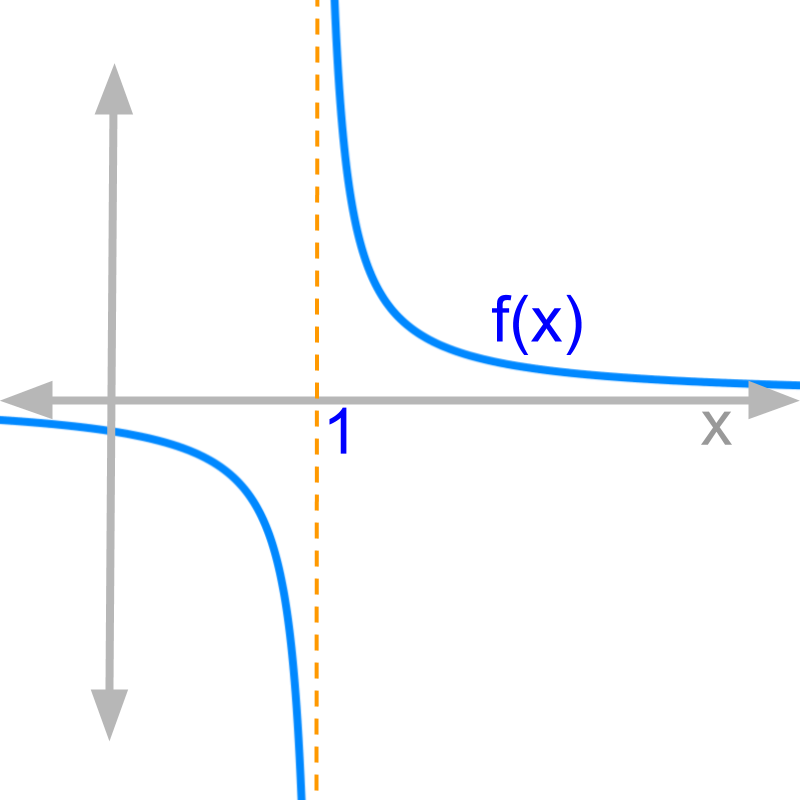maths > calculus-limits

Continuity of a Function at an input value

what you'll learn...

Value of a function

»  Value of f(x)$f \left(x\right)$
→  Evaluated at input f(x)x=a$f \left(x\right) {|}_{x = a}$ or f(a)$f \left(a\right)$
→  Left-hand-limit limxaf(x)${\lim}_{x \to a -} f \left(x\right)$
→  Right-hand-limit limxa+f(x)${\lim}_{x \to a +} f \left(x\right)$

»  A function f(x)$f \left(x\right)$ at $x = a$ is
→  continuous: if $f \left(a\right)$ = LHL = RHL
→  defined by value: if $f \left(a\right)$ is a real number
→  defined by limit: if $f \left(a\right) = \frac{0}{0}$ and LHL = RHL
→  not defined: if LHL $\ne$ RHL and $f \left(a\right) \notin \mathbb{R}$

Limit of a Function

»  If both left-hand-limit and right-hand-limit are equal, it is together referred as "limit of the function"

another motivation

So far, the motivation to examine limits of a function was to evaluate the function at a input value of the argument variable where the function evaluates to 'indeterminate value'.
In this topic, another motivation to examine limits is explained.

Consider $f \left(x\right) = \frac{1}{\textcolor{c \mathmr{and} a l}{x - 1}}$ at $x = 1$

by directly substituting $x = 1$
$f \left(1\right) = \frac{1}{1 - 1} = + \infty$

${\lim}_{x \to 1 +} f \left(x\right)$
$\quad \quad = \frac{1}{\textcolor{c \mathmr{and} a l}{1 + \delta - 1}}$
$\quad \quad = \frac{1}{\textcolor{c \mathmr{and} a l}{\delta}}$
$\quad \quad = \frac{1}{0} = + \infty$

${\lim}_{x \to 1 -} f \left(x\right)$
$\quad \quad = \frac{1}{\textcolor{c \mathmr{and} a l}{1 - \delta - 1}}$
$\quad \quad = \frac{1}{\textcolor{c \mathmr{and} a l}{- \delta}}$
$\quad \quad = - \frac{1}{0} = - \infty$.For the function $f \left(x\right) = \frac{1}{\textcolor{c \mathmr{and} a l}{x - 1}}$,
•  $f \left(a\right) = \infty$
•  ${\lim}_{x \to 1 +} f \left(x\right) = \infty$
•  ${\lim}_{x \to 1 -} f \left(x\right) = - \infty$

The plot of the function is given in the figure.

That is, for a value less than $x = 1$, the function is $- \infty$. And at $x = 1$, the function becomes $\infty$.

The function is not continuous.

continuous

A function $f \left(x\right)$ at a given input value $x = a$ is continuous if all the three are equal
$f \left(x\right) {|}_{x = a}$
$\quad \quad = {\lim}_{x \to a -} f \left(x\right)$
$\quad \quad = {\lim}_{x \to a +} f \left(x\right)$

The word 'continuous' means: unbroken and continue from one side to another without pause in between.

A function is continuous at an input value, if the following three are equal

•   function evaluated at the input

•   left-hand limit of the function at that input value and

•   right-hand limit of the function at that input value.

example

Given function $f \left(x\right) = 2 {x}^{2}$, is it continuous at x=0?

The answer is 'Yes, Continuous'. Evaluate the three values of the function and they are equal.

summary

Continuity of a Function: A function $f \left(x\right)$ is continuous at $x = a$ if all the following three have a defined value and are equal

•  Evaluated at the input value $f \left(x\right) {|}_{x = a}$

•  left-hand-limit ${\lim}_{x \to a -} f \left(x\right)$

•  right-hand-limit ${\lim}_{x - a +} f \left(x\right)$

limit of a function

Given that function $f \left(x\right)$ evaluates to indeterminate value at $x = a$. To evaluate the expected value of $f \left(x\right) {|}_{x = a}$, we examine ;

•  Left-hand-limit ${\lim}_{x \to a -} f \left(x\right)$

•  Right-hand-limit ${\lim}_{x \to a +} f \left(x\right)$

If these two limits are equal then the result is referred as "limit of the function at the input value" ${\lim}_{x \to a} f \left(x\right)$

The significance of this is that, most functions have both right-hand-limit and left-hand-limit equal.

summary

Limit of a function: Given function $f \left(x\right)$ and that $f \left(x\right) {|}_{x = a} = \frac{0}{0}$.
If ${\lim}_{x \to a +} f \left(x\right) = {\lim}_{x \to a -} f \left(x\right)$,
then the common value is referred as limit of the function ${\lim}_{x \to a} f \left(x\right)$.

discontinuous

If a function $f \left(x\right)$ is discontinuous at $x = a$, then what is ${\lim}_{x \to a} f \left(x\right)$?

The answer is 'cannot be computed'. It is given that the function is discontinuous at $x = a$, and that implies left-hand-limit and right-hand-limits are not equal. In that case, limit of the function cannot be computed without specifying left or right.

summary

Continuity of a Function: A function $f \left(x\right)$ is continuous at $x = a$ if all the following three have a defined value and are equal

•  Evaluated at the input value $f \left(x\right) {|}_{x = a}$

•  left-hand-limit ${\lim}_{x \to a -} f \left(x\right)$

•  right-hand-limit ${\lim}_{x - a +} f \left(x\right)$

Limit of a function: Given function $f \left(x\right)$ and that $f \left(x\right) {|}_{x = a} = \frac{0}{0}$.
If ${\lim}_{x \to a +} f \left(x\right) = {\lim}_{x \to a -} f \left(x\right)$,
then the common value is referred as limit of the function ${\lim}_{x \to a} f \left(x\right)$

Outline